## Example Questions

← Previous 1

### Example Question #1 : How To Add Exponents

For all x, 2x2 times 12x3 equals...

0

24x5

14x5

14x6

24x6

24x5

Explanation:

You multiply the integers, then add the exponents on the x's, giving you 24x5.

### Example Question #2 : How To Add Exponents

Multiply: 2x² * 3x

6x²

2x3

6x3

5x

5x3

6x3

Explanation:

When multiplying exponents you smiply add the exponents.

For 2x² times 3x, 2 times 3 is 6, and 2 + 1 is 3, so 2x² times 3x = 6x3

### Example Question #1 : How To Add Exponents

What is 23 + 22 ?

20

64

12

32

12

Explanation:

Using the rules of exponents, 23 + 22 = 8 + 4 = 12

### Example Question #4 : How To Add Exponents

Solve forwhere: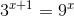1

5

9

2

3

1

Explanation:

The only value of x where the two equations equal each other is 1. All you have to do is substitute the answer choices in for x.

### Example Question #5 : How To Add Exponents

A particle travels 9 x 107 meters per second in a straight line for 12 x 10-6 seconds. How many meters has it traveled?

1.08 x 103

1.08 x 10

1.08

1.08 x 105

1.08 x 103

Explanation:

Multiplying the two numbers yields 1080. Expressed in scientific notation 1080 is 1.08 x 103.

### Example Question #1 : How To Add Exponents

Simplify: hn + h–2n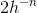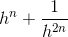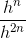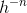Explanation:

h–2n = 1/h2n

hn + h–2n = hn + 1/h2n

### Example Question #7 : How To Add Exponents

Simplify: 3y2 + 7y2 + 9y3 – y3 + y

10y2 + 8y3 + y

10y4 + 8y6 + y

10y2 + 10y3 + y

19y11

10y2 + 9y3

10y2 + 8y3 + y

Explanation:

Add the coefficients of similar variables (y, y2, 9y3)

3y2 + 7y2 + 9y3 – y3 + y =

(3 + 7)y2 + (9 – 1)y3 + y =

10y2 + 8y3 + y

### Example Question #1 : How To Add Exponents

Simplify the following: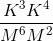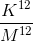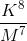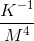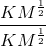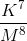Explanation:

When common variables have exponents that are multiplied, their exponents are added. So K* K4 =K(3+4) = K7.  And M6 * M2 = M(6+2) = M8. So the answer is K7/M8.

### Example Question #9 : How To Add Exponents

Solve for: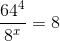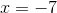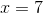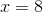Explanation:

First, reduce all values to a common base using properties of exponents.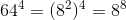Plugging back into the equation-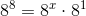Using the formula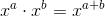We can reduce our equation to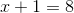So,### Example Question #81 : Exponents

Simplify:  y3x4(yx3 + y2x2 + y15 + x22)

y3x12 + y6x8 + y45 + x88

2x4y4 + 7y15 + 7x22

y3x12 + y12x8 + y24x4 + y3x23

y4x7 + y5x6 + y18x4 + y3x26

y3x12 + y6x8 + y45x4 + y3x88